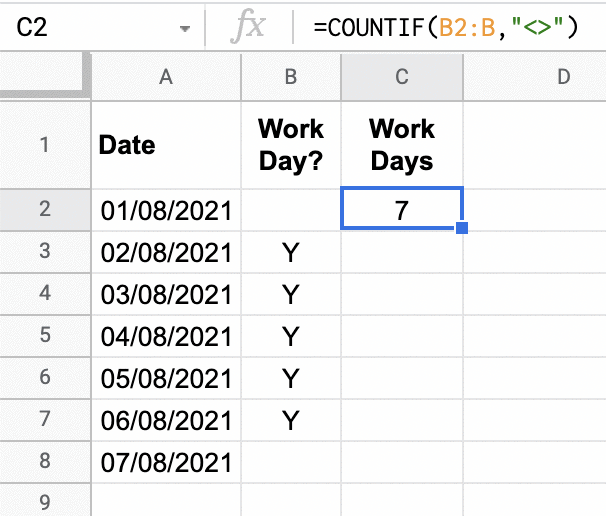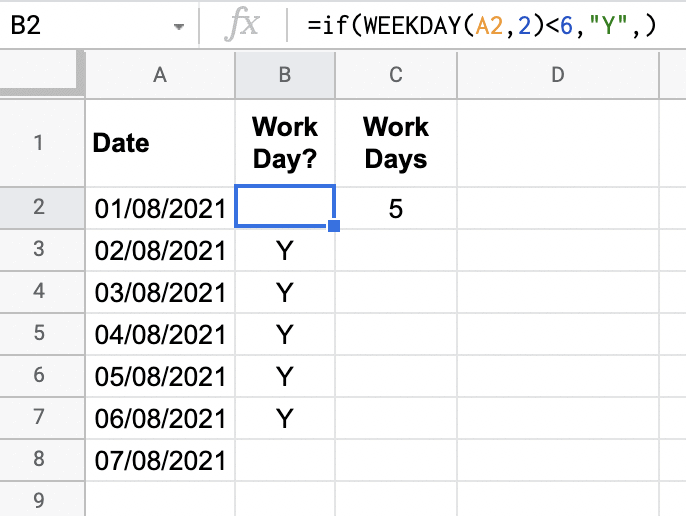# How Do I COUNTIF Non-Blank Cells In Google Sheets?The ``` COUNTIF ``` function in Google Sheets is a simple function which allows for the user to count a range of cells according to one specific filter. If more filters are required for a range, then ``` COUNTIFS ``` would be used instead.

What, though, if the simple criteria to apply to my count is to count all cells that are not blank? How do I annotate in the criterion of the ``` COUNTIF ``` functions to only count NON-blank cells?

To count only non-blank cells in a range, in the criterion parameter of a ``` COUNTIF ``` or ``` COUNTIFS ``` formula use the expression of not blank as ``` "<>" ``` . For example, to count values in ``` range ``` that are not blank write the formula as: ``` =COUNTIF(range,"<>") ``` , or if applying multiple criteria where the check for a range not being blank is just one condition use ``` =COUNTIFS(range1,"<>",...) ```

## Does ``` COUNTIF ``` Count Blank Cells?

Sometimes the ``` COUNTIF ``` function can appear to be providing the incorrect result, even though the formula specifically states to only count NON-blank cells.

Why then would it be counting blank cells?

Have a look at the following example:

It can seem like there’s an error with the ``` COUNTIF ``` function counting only non-blank cells, but there’s a very important thing not evident in the way the “Work Day?” column reports it’s data. It itself is a function written as so (for cell B2):

``=IF(WEEKDAY(A2,2)<6,"Y","")``

This formula above stating: if the value in the Date column is a weekend (1 = Monday, 2 = Tuesday, etc) then enter the string ``` "Y" ``` , otherwise enter a blank string ``` "" ``` .

If we changed this formula slightly to the following, we get a very different ``` COUNTIF ``` result:

``=IF(WEEKDAY(A2,2)<6,"Y",)``

Notice we don’t return anything if it is a weekend date? What result do we get?Notice the change in Work Day formula, provides now a correct result in our COUNTIF function!

In summary, COUNTIF will count blank cells if these are empty string “” cells, in other words they have the appearance of being “blank” (empty), but in fact are not. Therefore, take consideration to any formulas where a result is returning an empty string and whether this is intended, or if the result actually needs to be genuinely blank.

## Summary

To apply a filter in either the ``` COUNTIF ``` or ``` COUNTIFS ``` functions to count cells that are not blank, use the filter expression ``` "<>" ``` in the criterion parameters for each formula. For example, ``` =COUNTIF(Range, "<>") ``` or ``` =COUNTIFS(Range1, "<>", Range2, "<>") ``` .

Be careful with formulas returning an empty string ( ``` "" ``` ) as this will be seen as a NON-blank cell and will display an unexpected result with ``` COUNTIF ``` and ``` COUNTIFS ``` formulas.

Author of scripteverything.com, Ryan has been dabbling in code since the late '90s when he cut his teeth by exploring VBA in Excel when trying to do something more. Having his eyes opened with the potential of automating repetitive tasks, he expanded to Python and then moved over to scripting languages such as HTML, CSS, Javascript and PHP. When he is not behind a screen, Ryan enjoys a good bush walk with the family during the cooler months, and going with them to the beach during the warmer months.